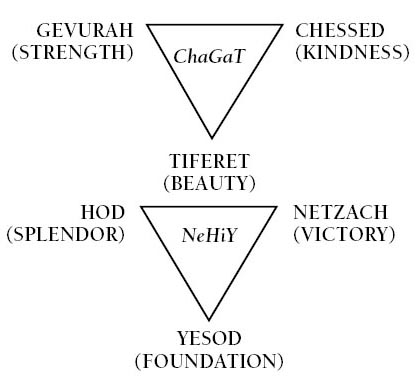We all know that we leave in a three-dimensional world. Up-down, right-left, forward-backward – these are well-familiar to us directions in three dimensions of space we occupy. However, we also live in a three-dimensional world of another kind. In physics, we have units of measurement. There are lots of them, but only three are fundamental – all other are various combinations of these three – meter, second and kilogram (yes, in science, we use metric rather than Imperial units). We measure space in meters (m), we measure time in seconds (s) and we measure mass in kilograms (kg). These three units are fundamental in a sense that they are irreducible and all other units are combinations of meter (m), second (s) and kilogram (kg) (please note that in this context, kilogram is not a measure of weight, but a measure of mass, which are not quite the same). For example, force is measured in Newtons  (m·kg/s2), energy is measured in Joules (1 Joule = 1 Newton · 1 meter – m2·kg/s2), Watt is Joule per second (m2·kg/s3,), etc.

These three units form a three-dimensional space, where X dimension is a space per se, Y dimension is time and Z dimension is mass. Any particle having mass m0, which is, at time t0, is in point A can be said to occupy a point in this three-dimensional space having coordinates (m0, t0, A). Needless to say, that point A is itself a different three-dimensional space – well-familiar to us space of up-down, right-left, forward-backward, where each point A is a set of three numbers (x, y, z). The second space is included in the first. One can visualize this by imagining that each point A is replaced by a three-dimensional balloon.

Space, time, and mass are thought to be completely independent from each other. But is it really so? Let’s consider the essence of each of these concepts.

What is time? Aristotle already noted that time, at its essence, is change (or the rate of change).

Space, on the other hand, is the opposite of time – at its essence, it’s the notion of stability, lack of change. Space is static. It is the backdrop of the dynamical world that plays out on the background of space. It is the canvas on which this world is painted. In short, space is the absence of change.

What is mass? Mass measures the resistance of a body to change. Recall the Second Law of Newton – acceleration is proportional to force acting on a body – a·m = F, where a is acceleration, m is mass and F is the force. Or, a = 1/m · F. We see that given certain F, the greater is the mass m the less is acceleration a. In other words, mass is the measure of resistance to change.

There you have it: we just reduced all three seemingly independent concepts to one fundamental notion of change – time is change, space is the absence of chance, and mass is the resistance to change.

Let’s now switch gears and consider the spiritual world with its sefirotic structure. Sefirotic tree is made of three triads – Chochmah-Binah-Daat, Chesed-Gevurah-Tiferet and Netzach-Chod-Yesod plus Malchut. Where do this triads come from? In his commentary on Sefer Yetzirah, Rabbi Ariyeh Kaplan suggests that they come from a philosophical triad – Thesis-Antithesis-Synthesis.  For example, is Chesed is a thesis, Gevurah on the left is the antithesis of Chesed on the right, and Tifere in the middle is the synthesis of Chesed and Gevurah.

Interestingly, the triad of Space-Time-Mass also seems to fall neatly into the primordial triad of Thesis-Antithesis-Synthesis. Indeed, if time (change) is a thesis, then space (the absence of change) is the antithesis. Mass that is connected with both as the resistance to change is a synthesis of time and space. We see here how spiritual concepts, sefirotic triads, such as Chesed, Gevurah and Tiferet, dress in the material world in physical characteristics of Time, Space and Mass.